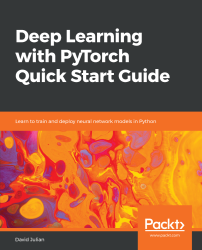•#### Deep Learning with PyTorch Quick Start Guide#### Overview of this book

PyTorch is extremely powerful and yet easy to learn. It provides advanced features, such as supporting multiprocessor, distributed, and parallel computation. This book is an excellent entry point for those wanting to explore deep learning with PyTorch to harness its power. This book will introduce you to the PyTorch deep learning library and teach you how to train deep learning models without any hassle. We will set up the deep learning environment using PyTorch, and then train and deploy different types of deep learning models, such as CNN, RNN, and autoencoders. You will learn how to optimize models by tuning hyperparameters and how to use PyTorch in multiprocessor and distributed environments. We will discuss long short-term memory network (LSTMs) and build a language model to predict text. By the end of this book, you will be familiar with PyTorch's capabilities and be able to utilize the library to train your neural networks with relative ease.
PrefaceFree Chapter
Introduction to PyTorchDeep Learning FundamentalsComputational Graphs and Linear ModelsConvolutional NetworksOther NN ArchitecturesGetting the Most out of PyTorchOther Books You May Enjoy# Benchmarking models

Benchmarking and evaluation are core to the success of any deep learning exploration. We will develop some simple code to evaluate two key performance measures: the accuracy and the training time. We will use the following model template:This model is the most common and basic linear template for solving MNIST. You can see we initialize each layer, in theinit method, by creating a class variable that is assigned to a PyTorch nn object. Here, we initialize two linear functions and a ReLU function. The nn.Linear function takes an input size of 28*28 or 784. This is the size of each of the training images. The output channels or the width of the network are set to 100. This can be set to anything, and in general a higher number will give better performance within the constraints of computing resources and the tendency for wider networks to overfit training data...